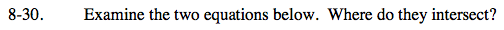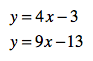### Home > CAAC > Chapter 8 > Lesson 8.1.3 > Problem8-30

8-30.One strategy is to set the equations equal to each other to solve for x.

4x − 3 = 9x − 13
10 = 5x
2 = x

Now substitute x back into one of the original equations to solve for y.

y = 4(2) − 3
y = 8 − 3
y = 5

The two lines intersect at (2, 5).